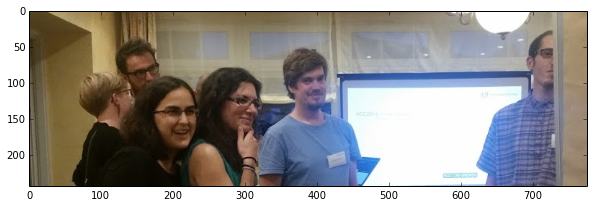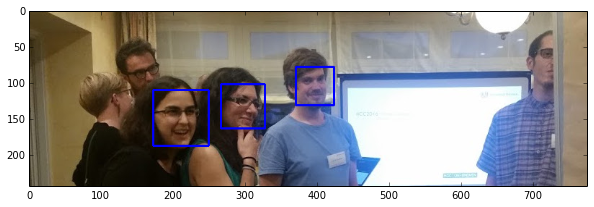## Detecting specific things (like faces)

One of the key things we can do with vision is detect specifics. Faces, humans...

The Viola Jones face detector is still a really well respected detector, and that's the one we'll use for our OpenCV face detection.

In :
# these imports let you use opencv
import cv2 #opencv itself
import common #some useful opencv functions
import video # some video stuff
import numpy as np # matrix manipulations

#the following are to do with this interactive notebook code
%matplotlib inline
from matplotlib import pyplot as plt # this lets you draw inline pictures in the notebooks
import pylab # this allows you to control figure size
pylab.rcParams['figure.figsize'] = (10.0, 8.0) # this controls figure size in the notebook
In :
face_image = cv2.imread('test.jpg')
grey = cv2.cvtColor(img, cv2.COLOR_BGR2GRAY)
plt.imshow(cv2.cvtColor(face_image, cv2.COLOR_BGR2RGB))

Out:
<matplotlib.image.AxesImage at 0x7f8f8fa64190>In :
# this is a pre-trained face cascade
face_cascade = cv2.CascadeClassifier('haarcascade_frontalface_default.xml')
faces = face_cascade.detectMultiScale(grey, 1.3, 5)
for (x,y,w,h) in faces:
cv2.rectangle(face_image,(x,y),(x+w,y+h),(255,0,0),2)
plt.imshow(cv2.cvtColor(face_image, cv2.COLOR_BGR2RGB))

Out:
<matplotlib.image.AxesImage at 0x7f8f8c0679d0>In [ ]: